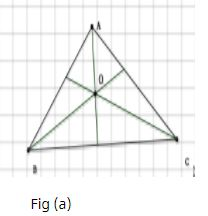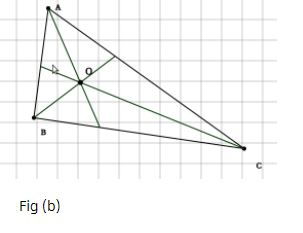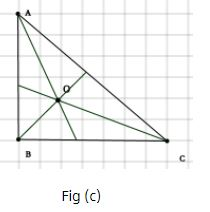# Procedure - To illustrate that the Internal Bisectors of the Angles of a Triangle Concur at a Point Class 9 Notes | EduRev

## Class 9 : Procedure - To illustrate that the Internal Bisectors of the Angles of a Triangle Concur at a Point Class 9 Notes | EduRev

The document Procedure - To illustrate that the Internal Bisectors of the Angles of a Triangle Concur at a Point Class 9 Notes | EduRev is a part of the Class 9 Course Mathematics (Maths) Class 9.
All you need of Class 9 at this link: Class 9

As performed in real lab:

Material required:

Coloured papers, fevicol and a pair of scissors.

Procedure:

1. Cut an acute angled triangle from a colored paper and name it as ABC.

2. Fold along the vertex A of the triangle in such a way that the side AB lies along AC.

3. The crease thus formed is the angle bisector of angle A. Similarly, get the angle bisectors of angle B and C.   [Fig (a)].

4. Repeat the same activity for a obtuse angled triangle and right angled triangle. [Fig (b) and  (c)].

As performed in the simulator:

1.Select three points A, B and C anywhere on the workbench  to draw a triangle.

2. Depending on your points selection acute, obtuse or right angled triangle is drawn.

3. Now, click on each vertex of the triangle to draw its angle bisector. You can use the protractor to measure the angles .

4.Activity completed successfully. You can see the inference below.Observations:

•  We see that the three angle bisectors are concurrent and the point is called the incentre (O).

•  We observe that the incentre of an acute, an obtuse and right angled triangle always lies inside the  triangle.

Offer running on EduRev: Apply code STAYHOME200 to get INR 200 off on our premium plan EduRev Infinity!

## Mathematics (Maths) Class 9

190 videos|233 docs|82 tests

,

,

,

,

,

,

,

,

,

,

,

,

,

,

,

,

,

,

,

,

,

;